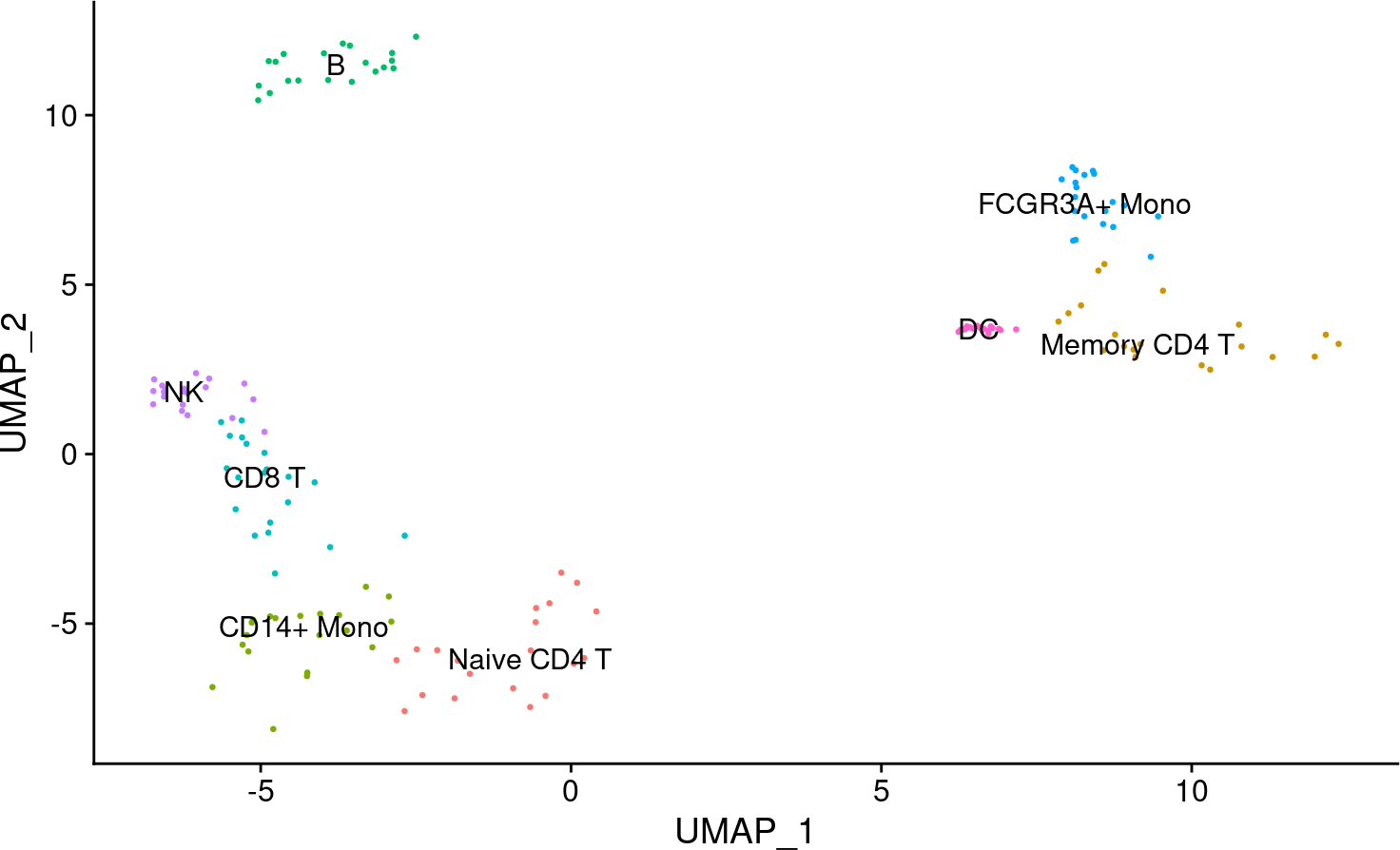scRNA-seq yield many molecular readouts that are hard to interpret by themselves. One way of summarizing this information is by inferring transcription factor (TF) activities from prior knowledge.

In this notebook we showcase how to use `decoupleR` for transcription factor activity inference with a down-sampled PBMCs 10X data-set. The data consists of 160 PBMCs from a Healthy Donor. The original data is freely available from 10x Genomics here from this webpage.

First, we need to load the relevant packages, `Seurat` to handle scRNA-seq data and `decoupleR` to use statistical methods.

``````## We load the required packages
library(Seurat)
library(decoupleR)

# Only needed for data handling and plotting
library(dplyr)
library(tibble)
library(tidyr)
library(patchwork)
library(ggplot2)
library(pheatmap)``````

Here we used a down-sampled version of the data used in the `Seurat` vignette. We can open the data like this:

``````inputs_dir <- system.file("extdata", package = "decoupleR")

We can observe that we have different cell types:

``DimPlot(data, reduction = "umap", label = TRUE, pt.size = 0.5) + NoLegend()``# 3 DoRothEA network

DoRothEA is a comprehensive resource containing a curated collection of TFs and their transcriptional targets. Since these regulons were gathered from different types of evidence, interactions in DoRothEA are classified in different confidence levels, ranging from A (highest confidence) to D (lowest confidence). Moreover, each interaction is weighted by its confidence level and the sign of its mode of regulation (activation or inhibition).

For this example we will use the human version (mouse is also available) and we will use the confidence levels ABC. We can use `decoupleR` to retrieve it from `OmniPath`:

``````net <- get_dorothea(organism='human', levels=c('A', 'B', 'C'))
net
#> # A tibble: 32,277 × 4
#>    source confidence target     mor
#>    <chr>  <chr>      <chr>    <dbl>
#>  1 ADNP   C          ATF7IP   0.333
#>  2 ADNP   C          DYRK1A   0.333
#>  3 ADNP   C          TLK1     0.333
#>  4 ADNP   C          ZMYM4    0.333
#>  5 AHR    C          ARHGAP15 0.333
#>  6 AHR    C          ARID5B   0.333
#>  7 AHR    B          ASAP1    0.5
#>  8 AHR    C          CREB5    0.333
#>  9 AHR    C          CTNNA1   0.333
#> 10 AHR    C          CTNNA2   0.333
#> # … with 32,267 more rows``````

# 4 Activity inference with Weighted Mean

To infer activities we will run the Weighted Mean method (`wmean`). It infers regulator activities by first multiplying each target feature by its associated weight which then are summed to an enrichment score `wmean`. Furthermore, permutations of random target features can be performed to obtain a null distribution that can be used to compute a z-score `norm_wmean`, or a corrected estimate `corr_wmean` by multiplying `wmean` by the minus log10 of the obtained empirical p-value.

In this example we use `wmean` but we could have used any other. To see what methods are available use `show_methods()`.

To run `decoupleR` methods, we need an input matrix (`mat`), an input prior knowledge network/resource (`net`), and the name of the columns of net that we want to use.

``````# Extract the normalized log-transformed counts
mat <- as.matrix(data@assays\$RNA@data)

# Run wmean
acts <- run_wmean(mat=mat, net=net, .source='source', .target='target',
.mor='mor', times = 100, minsize = 5)
acts
#> # A tibble: 131,520 × 5
#>    statistic  source condition         score p_value
#>    <chr>      <chr>  <chr>             <dbl>   <dbl>
#>  1 corr_wmean AHR    AAACATACAACCAC-1 0.947     0.02
#>  2 corr_wmean AHR    AAACGCTGTTTCTG-1 0.203     0.24
#>  3 corr_wmean AHR    AACCTTTGGACGGA-1 1.49      0.02
#>  4 corr_wmean AHR    AACGCCCTCGTACA-1 0.0682    0.56
#>  5 corr_wmean AHR    AACGTCGAGTATCG-1 0.0285    0.36
#>  6 corr_wmean AHR    AACTCACTCAAGCT-1 0.389     0.14
#>  7 corr_wmean AHR    AAGATGGAAAACAG-1 0.173     0.24
#>  8 corr_wmean AHR    AAGATTACCGCCTT-1 1.32      0.02
#>  9 corr_wmean AHR    AAGCCATGAACTGC-1 0.560     0.08
#> 10 corr_wmean AHR    AAGGTCTGCAGATC-1 0.149     0.28
#> # … with 131,510 more rows``````

# 5 Visualization

From the obtained results, we will select the `norm_wmean` activities and store them in our object as a new assay called `tfswmean`:

``````# Extract norm_wmean and store it in tfswmean in pbmc
data[['tfswmean']] <- acts %>%
filter(statistic == 'norm_wmean') %>%
pivot_wider(id_cols = 'source', names_from = 'condition',
values_from = 'score') %>%
column_to_rownames('source') %>%
Seurat::CreateAssayObject(.)

# Change assay
DefaultAssay(object = data) <- "tfswmean"

# Scale the data
data <- ScaleData(data)
data@assays\$tfswmean@data <- data@assays\$tfswmean@scale.data``````

This new assay can be used to plot activities. Here we observe the activity inferred for PAX5 across cells, which it is particulary active in B cells. Interestingly, PAX5 is a known TF crucial for B cell identity and function. The inference of activities from “foot-prints” of target genes is more informative than just looking at the molecular readouts of a given TF, as an example here is the gene expression of PAX5, which is not very informative by itself:

``````p1 <- DimPlot(data, reduction = "umap", label = TRUE, pt.size = 0.5) +
NoLegend() + ggtitle('Cell types')
p2 <- (FeaturePlot(data, features = c("PAX5")) &
scale_colour_gradient2(low = 'blue', mid = 'white', high = 'red')) +
ggtitle('PAX5 activity')
DefaultAssay(object = data) <- "RNA"
p3 <- FeaturePlot(data, features = c("PAX5")) + ggtitle('PAX5 expression')
DefaultAssay(object = data) <- "tfswmean"
p1 | p2 | p3``````